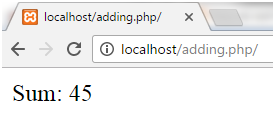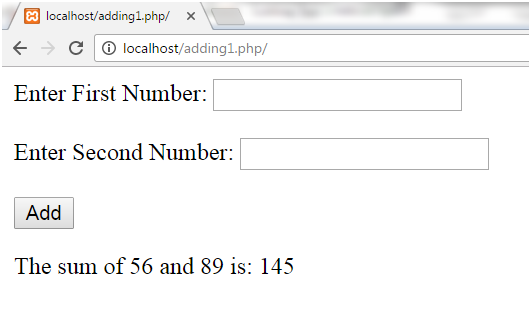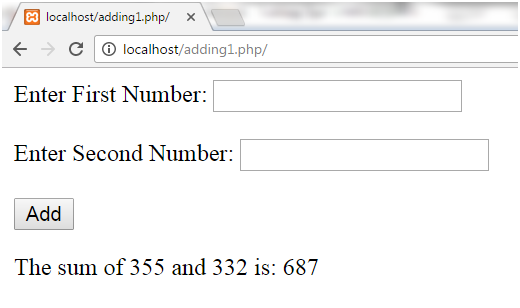Q:

# Adding Two Numbers in Php

There are three methods to add two numbers:

• Adding in simple code in PHP
• Adding in form in PHP
• Adding without using arithmetic operator (+).

Addition of two numbers 15 and 30 is shown here.

Example:

``````<?php
\$x=15;
\$y=30;
\$z=\$x+\$y;
echo "Sum: ",\$z;
?>  ``````

Output:Two numbers can be added by passing input value in the form.

Example:

``````<html>
<body>
<form method="post">
Enter First Number:
<input type="number" name="number1" /><br><br>
Enter Second Number:
<input type="number" name="number2" /><br><br>
</form>
<?php
if(isset(\$_POST['submit']))
{
\$number1 = \$_POST['number1'];
\$number2 = \$_POST['number2'];
\$sum =  \$number1+\$number2;
echo "The sum of \$number1 and \$number2 is: ".\$sum;
}
?>
</body>
</html>  ``````

Output:Two numbers can be added by passing input value in the form but without using (+) operator.

``````<body>
<form>
Enter First Number:
<input type="number" name="number1" /><br><br>
Enter Second Number:
<input type="number" name="number2" /><br><br>
</form>
</body>
<?php
@\$number1=\$_GET['number1'];
@\$number2=\$_GET['number2'];
for (\$i=1; \$i<=\$number2; \$i++)
{
\$number1++;
}
echo "Sum of \$number1 and \$number2 is=".\$number2;
?>  ``````

Output: